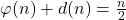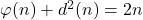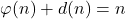# On certain equations and inequalities involving the arithmetical functions φ(n) and d(n)

József Sándor and Saunak Bhattacharjee
Notes on Number Theory and Discrete Mathematics
Print ISSN 1310–5132, Online ISSN 2367–8275
Volume 28, 2022, Number 2, Pages 376–379
DOI: 10.7546/nntdm.2022.28.2.376-379

## Details

### Authors and affiliations

József SándorBabeș-Bolyai University, Department of Mathematics
Cluj-Napoca, Romania

Saunak BhattacharjeeIndian Institute of Science Education and Research
Tirupati, India

### Abstract

By using the results and methods of , we will study the equationand the related inequalities. The equationwill be solved, too.

### Keywords

• Arithmetic functions
• Inequalities

• 11A25

### References

1. Sándor, J. (2020). On the equationand related inequalities. Notes on Number Theory and Discrete Mathematics, 26(3), 1–4.
2. Sándor, J., & Atanassov, K. T. (2021). Arithmetic Functions. Nova Science Publishers, New York.
3. Sándor, J., & Kovács, L. (2009). An inequality for the number of divisors. Octogon Mathematical Magazine, 17(2), 746–749.
4. Sándor, J., Mitrinović, D. S., & Crstici, B. (2005). Handbook of Number Theory I. Springer.